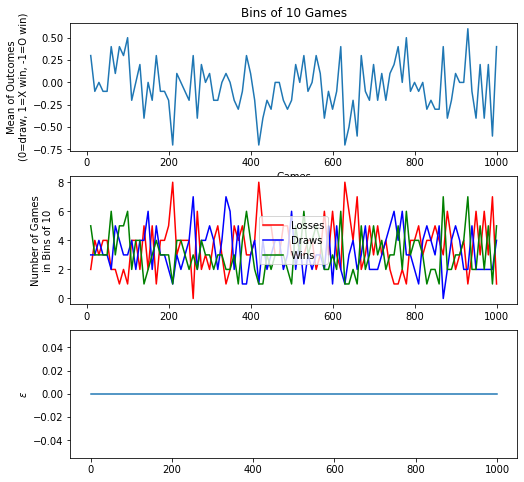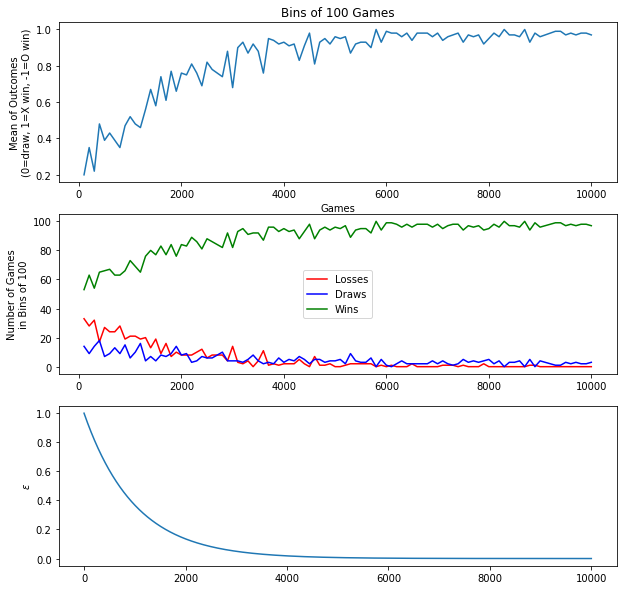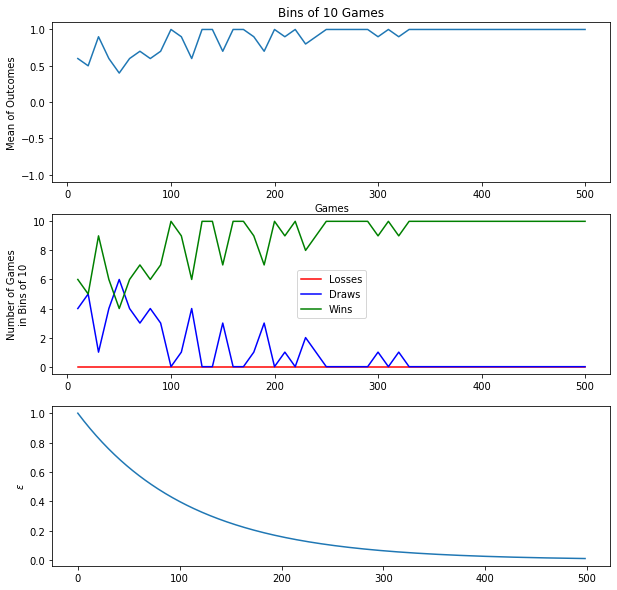$$\newcommand{\Rv}{\mathbf{R}} \newcommand{\rv}{\mathbf{r}} \newcommand{\Qv}{\mathbf{Q}} \newcommand{\Qnv}{\mathbf{Qn}} \newcommand{\Av}{\mathbf{A}} \newcommand{\Aiv}{\mathbf{Ai}} \newcommand{\av}{\mathbf{a}} \newcommand{\xv}{\mathbf{x}} \newcommand{\Xv}{\mathbf{X}} \newcommand{\yv}{\mathbf{y}} \newcommand{\Yv}{\mathbf{Y}} \newcommand{\zv}{\mathbf{z}} \newcommand{\av}{\mathbf{a}} \newcommand{\Wv}{\mathbf{W}} \newcommand{\wv}{\mathbf{w}} \newcommand{\betav}{\mathbf{\beta}} \newcommand{\gv}{\mathbf{g}} \newcommand{\Hv}{\mathbf{H}} \newcommand{\dv}{\mathbf{d}} \newcommand{\Vv}{\mathbf{V}} \newcommand{\vv}{\mathbf{v}} \newcommand{\Uv}{\mathbf{U}} \newcommand{\uv}{\mathbf{u}} \newcommand{\tv}{\mathbf{t}} \newcommand{\Tv}{\mathbf{T}} \newcommand{\TDv}{\mathbf{TD}} \newcommand{\Tiv}{\mathbf{Ti}} \newcommand{\Sv}{\mathbf{S}} \newcommand{\Gv}{\mathbf{G}} \newcommand{\zv}{\mathbf{z}} \newcommand{\Zv}{\mathbf{Z}} \newcommand{\Norm}{\mathcal{N}} \newcommand{\muv}{\boldsymbol{\mu}} \newcommand{\sigmav}{\boldsymbol{\sigma}} \newcommand{\phiv}{\boldsymbol{\phi}} \newcommand{\Phiv}{\boldsymbol{\Phi}} \newcommand{\Sigmav}{\boldsymbol{\Sigma}} \newcommand{\Lambdav}{\boldsymbol{\Lambda}} \newcommand{\half}{\frac{1}{2}} \newcommand{\argmax}{\underset{#1}{\operatorname{argmax}}} \newcommand{\argmin}{\underset{#1}{\operatorname{argmin}}} \newcommand{\dimensionbar}{\underset{#1}{\operatorname{|}}} \newcommand{\grad}{\mathbf{\nabla}} \newcommand{\ebx}{e^{\betav_{#1}^T \xv_n}} \newcommand{\eby}{e^{y_{n,#1}}} \newcommand{\Tiv}{\mathbf{Ti}} \newcommand{\Fv}{\mathbf{F}} \newcommand{\ones}{\mathbf{1}_{#1}}$$

# Reinforcement Learning for Two-Player Games¶

How does Tic-Tac-Toe differ from the maze problem?

• Different state and action sets.
• Two players rather than one.
• Reinforcement is 0 until end of game, when it is 1 for win, 0 for draw, or -1 for loss.
• Maximizing sum of reinforcement rather than minimizing.
• Anything else?

## Representing the Q Table¶

The state is the board configuration. There are $3^9$ of them, though not all are reachable. Is this too big?

It is a bit less than 20,000. Not bad. Is this the full size of the Q table?

No. We must add the action dimension. There are at most 9 actions, one for each cell on the board. So the Q table will contain about $20,000 \cdot 9$ values or about 200,000. No worries.

Instead of thinking about the Q table as a three-dimensional array, as we did last time, let's be more pythonic and use a dictionary. Use the current state as the key, and the value associated with the state is an array of Q values for each action taken in that state.

We still need a way to represent a board.

How about an array of characters? So

 X |   | O
---------
| X | O
---------
X |   |



would be

 board = np.array(['X',' ','O', ' ','X','O', 'X',' ',' '])



The initial board would be

 board = np.array([' ']*9)



We can represent a move as an index, 0 to 8, into this array.

What should the reinforcement values be?

How about 0 every move except when X wins, with a reinforcement of 1, and when O wins, with a reinforcement of -1.

For the above board, let's say we, meaning Player X, prefer move to index 3. In fact, this always results in a win. So the Q value for move to 3 should be 1. What other Q values do you know?

If we don't play a move to win, O could win in one move. So the other moves might have Q values close to -1, depending on the skill of Player O. In the following discussion we will be using a random player for O, so the Q value for a move other than 8 or 3 will be close to but not exactly -1.

## Agent-World Interaction Loop¶

For our agent to interact with its world, we must implement

1. Initialize Q.
2. Set initial state, as empty board.
3. Repeat:
1. Agent chooses next X move.
2. If X wins, set Q(board,move) to 1.
3. Else, if board is full, set Q(board,move) to 0.
4. Else, let O take move.
5. If O won, update Q(board,move) by (-1 - Q(board,move))
6. For all cases, update Q(oldboard,oldmove) by Q(board,move) - Q(oldboard,oldmove)
7. Shift current board and move to old ones.

## Now in Python¶

First, here is the result of running tons of games.First, let's get some function definitions out of the way.

In :
import numpy as np
import matplotlib.pyplot as plt
import copy


Let's write a function to print a board in the usual Tic-Tac-Toe style.

In :
def printBoard(board):
print('''
{}|{}|{}
-----
{}|{}|{}
------
{}|{}|{}'''.format(*tuple(board)))
printBoard(np.array(['X',' ','O', ' ','X','O', 'X',' ',' ']))

X| |O
-----
|X|O
------
X| |


Let's write a function that returns True if the current board is a winning board for us. We will be Player X. What does the value of combos represent?

In :
def winner(board):
combos = np.array((0,1,2, 3,4,5, 6,7,8, 0,3,6, 1,4,7, 2,5,8, 0,4,8, 2,4,6))
if np.any(np.logical_or(np.all('X' == board[combos].reshape((-1, 3)), axis=1),
np.all('O' == board[combos].reshape((-1, 3)), axis=1))):
return True
else:
return False

In :
board = np.array(['X',' ','O', ' ','X','O', 'X',' ',' '])
printBoard(board), print(winner(board))
board = np.array(['X',' ','X', ' ','X','O', 'X',' ',' '])
printBoard(board), print(winner(board))

X| |O
-----
|X|O
------
X| |
False

X| |X
-----
|X|O
------
X| |
True

Out:
(None, None)

How can we find all valid moves from a board? Just find all of the spaces in the board representation

In :
np.where(board == ' ')

Out:
(array([1, 3, 7, 8]),)
In :
np.where(board == ' ')

Out:
array([1, 3, 7, 8])

And how do we pick one at random and make that move?

In :
board = np.array(['X',' ','O', ' ','X','O', 'X',' ',' '])
validMoves = np.where(board == ' ')
move = np.random.choice(validMoves)
boardNew = copy.copy(board)
boardNew[move] = 'X'
print('From this board')
printBoard(board)
print('\n  Move',move)
print('\nresults in board')
printBoard(boardNew)

From this board

X| |O
-----
|X|O
------
X| |

Move 1

results in board

X|X|O
-----
|X|O
------
X| |


If X just won, we want to set the Q value for the previous state (board) to 1, because X will always win from that state and that action (move).

First we must figure out how to implement the Q table? We want to associate a value with each board and move. We can use a python dictionary for this. We know how to represent a board. A move can be an integer from 0 to 8 to index into the board array for the location to place a marker.

In :
Q = {}  # empty table
Q[(tuple(board), 1)] = 0
Q

Out:
{(('X', ' ', 'O', ' ', 'X', 'O', 'X', ' ', ' '), 1): 0}
In :
Q[(tuple(board), 1)]

Out:
0

What if we try to look up a Q value for a state,action we have not encountered yet? It will not be in the dictionary. We can use the get method for the dictionary, that has a second argument as the value returned if the key does not exist.

In :
board = 'X'
Q[(tuple(board), 1)]

---------------------------------------------------------------------------
KeyError                                  Traceback (most recent call last)
<ipython-input-13-f4e07b043832> in <module>
1 board = 'X'
----> 2 Q[(tuple(board),1)]

KeyError: (('X', 'X', 'O', ' ', 'X', 'O', 'X', ' ', ' '), 1)
In :
Q.get((tuple(board), 1), 42)

Out:
42

Now we can set the Q value for (board,move) to 1.

In :
Q[(tuple(board), move)] = 1


If the board is full, then the the previous state and action should be assigned 0.

In :
Q[(tuple(board), move)] = 0


If the board is not full, better check to see if O just won. If O did just win, then we should adjust the Q value of the previous state and X action to be closer to -1, because we just received a -1 reinforcement and the game is over.

In :
rho = 0.1 # learning rate
Q[(tuple(board), move)] += rho * (-1 - Q[(tuple(board), move)])


If nobody won yet, let's calculate the temporal difference error and use it to adjust the Q value of the previous board,move. We do this only if we are not at the first move of a game.

In :
step = 0
if step > 0:
Q[(tuple(boardOld), moveOld)] += rho * (Q[(tuple(board), move)] - Q[(tuple(boardOld), moveOld)])


Initially, taking random moves is a good strategy, because we know nothing about how to play Tic-Tac-Toe. But, once we have gained some experience and our Q table has acquired some good predictions of the sum of future reinforcement, we should rely on our Q values to pick good moves. For a given board, which move is predicted to lead to the best possible future using the current Q table?

In :
validMoves = np.where(board == ' ')
print('Valid moves are', validMoves)
Qs = np.array([Q.get((tuple(board), m), 0) for m in validMoves])
print('Q values for validMoves are', Qs)
bestMove = validMoves[np.argmax(Qs)]
print('Best move is', bestMove)

Valid moves are [3 7 8]
Q values for validMoves are [0 0 0]
Best move is 3


To slowly transition from taking random actions to taking the action currently believed to be best, called the greedy action, we slowly decay a parameter, $\epsilon$, from 1 down towards 0 as the probability of selecting a random action. This is called the $\epsilon$-greedy policy.

In :
s=np.array([4, 2, 5])
np.random.shuffle(s)
s

Out:
array([4, 2, 5])
In :
def epsilonGreedy(epsilon, Q, board):
validMoves = np.where(board == ' ')
if np.random.uniform() < epsilon:
# Random Move
return np.random.choice(validMoves)
else:
# Greedy Move
np.random.shuffle(validMoves)
Qs = np.array([Q.get((tuple(board) ,m), 0) for m in validMoves])
return validMoves[ np.argmax(Qs) ]

epsilonGreedy(0.8, Q, board)

Out:
3

Now write a function to make plots to show results of some games. Say the variable outcomes is a vector of 1's, 0's, and -1's, for games in which X wins, draws, and loses, respectively.

In :
outcomes = np.random.choice([-1, 0, 1], replace=True, size=(1000))
outcomes[:10]

Out:
array([ 1, -1,  0,  1,  0,  0,  1,  1,  1, -1])
In :
def plotOutcomes(outcomes, epsilons, maxGames, nGames):
if nGames == 0:
return
nBins = 100
nPer = maxGames // nBins
outcomeRows = outcomes.reshape((-1, nPer))
outcomeRows = outcomeRows[:nGames // nPer + 1, :]
avgs = np.mean(outcomeRows, axis=1)

plt.subplot(3, 1, 1)
xs = np.linspace(nPer, nGames, len(avgs))
plt.plot(xs, avgs)
plt.xlabel('Games')
plt.ylabel('Mean of Outcomes\n(0=draw, 1=X win, -1=O win)')
plt.title('Bins of {:d} Games'.format(nPer))

plt.subplot(3, 1, 2)
plt.plot(xs,np.sum(outcomeRows==-1, axis=1), 'r-', label='Losses')
plt.plot(xs,np.sum(outcomeRows==0,axis=1),'b-', label='Draws')
plt.plot(xs,np.sum(outcomeRows==1,axis=1), 'g-', label='Wins')
plt.legend(loc="center")
plt.ylabel('Number of Games\nin Bins of {:d}'.format(nPer))

plt.subplot(3, 1, 3)
plt.plot(epsilons[:nGames])
plt.ylabel('$\epsilon$')

In :
plt.figure(figsize=(8, 8))
plotOutcomes(outcomes, np.zeros(1000), 1000, 1000)Finally, let's write the whole Tic-Tac-Toe learning loop!

In :
from IPython.display import display, clear_output

In :
maxGames = 10000
rho = 0.5
epsilonDecayRate = 0.999
epsilon = 1.0
graphics = True
showMoves = not graphics

outcomes = np.zeros(maxGames)
epsilons = np.zeros(maxGames)
Q = {}

if graphics:
fig = plt.figure(figsize=(10, 10))

for nGames in range(maxGames):

epsilon *= epsilonDecayRate
epsilons[nGames] = epsilon
step = 0
board = np.array([' '] * 9)  # empty board
done = False

while not done:
step += 1

# X's turn
move = epsilonGreedy(epsilon, Q, board)
boardNew = copy.copy(board)
boardNew[move] = 'X'
if (tuple(board), move) not in Q:
Q[(tuple(board), move)] = 0  # initial Q value for new board,move
if showMoves:
printBoard(boardNew)

if winner(boardNew):
# X won!
if showMoves:
print('        X Won!')
Q[(tuple(board), move)] = 1
done = True
outcomes[nGames] = 1

elif not np.any(boardNew == ' '):
# Game over. No winner.
if showMoves:
print('        draw.')
Q[(tuple(board), move)] = 0
done = True
outcomes[nGames] = 0

else:
# O's turn.  O is a random player!
moveO = np.random.choice(np.where(boardNew==' '))
boardNew[moveO] = 'O'
if showMoves:
printBoard(boardNew)
if winner(boardNew):
# O won!
if showMoves:
print('        O Won!')
Q[(tuple(board), move)] += rho * (-1 - Q[(tuple(board), move)])
done = True
outcomes[nGames] = -1

if step > 1:
Q[(tuple(boardOld), moveOld)] += rho * (Q[(tuple(board), move)] - Q[(tuple(boardOld), moveOld)])

boardOld, moveOld = board, move # remember board and move to Q(board,move) can be updated after next steps
board = boardNew

if graphics and (nGames % (maxGames/10) == 0 or nGames == maxGames-1):
fig.clf()
plotOutcomes(outcomes, epsilons ,maxGames, nGames-1)
clear_output(wait=True)
display(fig);

if graphics:
clear_output(wait=True)
print('Outcomes: {:d} X wins {:d} O wins {:d} draws'.format(np.sum(outcomes==1), np.sum(outcomes==-1), np.sum(outcomes==0)))

Outcomes: 8958 X wins 556 O wins 486 drawsIn :
Q[(tuple([' ']*9),0)]

Out:
0.3530699169838406
In :
Q[(tuple([' ']*9),1)]

Out:
0.2877461808018128
In :
Q.get((tuple([' ']*9),0), 0)

Out:
0.3530699169838406
In :
[Q.get((tuple([' ']*9),m), 0) for m in range(9)]

Out:
[0.3530699169838406,
0.2877461808018128,
0.36035236644550817,
0.27268414988617473,
0.9858147425186672,
0.24873616345843108,
0.40305291938480975,
0.2824002335925688,
0.15765046968050456]
In :
board = np.array([' ']*9)
Qs = [Q.get((tuple(board),m), 0) for m in range(9)]
printBoard(board)
print('''{:.2f} | {:.2f} | {:.2f}
------------------
{:.2f} | {:.2f} | {:.2f}
------------------
{:.2f} | {:.2f} | {:.2f}'''.format(*Qs))

 | |
-----
| |
------
| |
0.35 | 0.29 | 0.36
------------------
0.27 | 0.99 | 0.25
------------------
0.40 | 0.28 | 0.16

In :
def printBoardQs(board,Q):
printBoard(board)
Qs = [Q.get((tuple(board),m), 0) for m in range(9)]
print()
print('''{:.2f} | {:.2f} | {:.2f}
------------------
{:.2f} | {:.2f} | {:.2f}
------------------
{:.2f} | {:.2f} | {:.2f}'''.format(*Qs))

In :
board = 'X'
board = 'O'
printBoardQs(board,Q)

X|O|
-----
| |
------
| |

0.00 | 0.00 | 0.00
------------------
0.88 | 0.25 | 0.00
------------------
0.00 | 0.00 | 0.00

In :
board = 'X'
board = 'O'
printBoardQs(board,Q)

X|O|
-----
O|X|
------
| |

0.00 | 0.00 | 0.25
------------------
0.00 | 0.00 | 0.00
------------------
0.00 | 0.00 | 0.00

In :
board = np.array([' ']*9)
printBoardQs(board,Q)

 | |
-----
| |
------
| |

0.35 | 0.29 | 0.36
------------------
0.27 | 0.99 | 0.25
------------------
0.40 | 0.28 | 0.16

In :
board = 'X'
board = 'O'
printBoardQs(board,Q)

X| |
-----
|O|
------
| |

0.00 | -0.25 | -0.17
------------------
-0.12 | 0.00 | -0.12
------------------
-0.25 | -0.25 | -0.25

In :
board = 'X'
board = 'O'
printBoardQs(board,Q)

X|O|X
-----
|O|
------
| |

0.00 | 0.00 | 0.00
------------------
-0.62 | 0.00 | -0.50
------------------
-0.32 | -0.38 | -0.34

In :
board = 'X'
board = 'O'
printBoardQs(board,Q)

X|O|X
-----
O|O|
------
|X|

0.00 | 0.00 | 0.00
------------------
0.00 | 0.00 | 0.00
------------------
-0.50 | 0.00 | -0.25

In :
board = 'X'
board = 'O'
printBoardQs(board,Q)

X|O|X
-----
O|O|X
------
O|X|

0.00 | 0.00 | 0.00
------------------
0.00 | 0.00 | 0.00
------------------
0.00 | 0.00 | 1.00


# Neural Network as Q function for Tic-Tac-Toe¶

In :
import neuralnetwork_regression as nn


To use a neural network, we must represent the board numerically. Let's use a vector of 9 values of either 1, -1, or 0 to represent 'X', 'O', or no markers.

And, let's use one Qnet for Player X and a different one for Player O!|

In :
def initial_state():
return np.array( * 9)

def next_state(s, a, marker):  # s is a board, and a is an index into the cells of the board, marker is 1 or -1
s = s.copy()
s[a] = 1 if marker == 'X' else -1
return s

def reinforcement(s):
if won('X', s):
return 1
if won('O', s):
return -1
return 0

def won(player, s):
marker = 1 if player == 'X' else -1
combos = np.array((0,1,2, 3,4,5, 6,7,8, 0,3,6, 1,4,7, 2,5,8, 0,4,8, 2,4,6))
return np.any(np.all(marker == s[combos].reshape((-1, 3)), axis=1))

def draw(s):
return sum(s == 0) == 0

In :
def valid_actions(state):
return np.where(state == 0)

In :
def stack_sa(s, a):
return np.hstack((s, a)).reshape(1, -1)

def other_player(player):
return 'X' if player == 'O' else 'O'

In :
def epsilon_greedy(Qnet, state, epsilon):

actions = valid_actions(state)

if np.random.uniform() < epsilon:
# Random Move
action = np.random.choice(actions)

else:
# Greedy Move
np.random.shuffle(actions)
Qs = np.array([Qnet.use(stack_sa(state, a)) for a in actions])
action = actions[np.argmax(Qs)]

return action

In :
def make_samples(Qnets, initial_state_f, next_state_f, reinforcement_f, epsilon):
'''Run one game'''
X = []
R = []
Qn = []

s = initial_state_f()
player = 'X'

while True:

a = epsilon_greedy(Qnets[player], s, epsilon)
sn = next_state_f(s, a, player)
r = reinforcement_f(s)

X.append(stack_sa(s, a))
R.append(r)

if r != 0 or draw(sn):
break

s = sn
player = other_player(player)  # switch

X = np.vstack(X)
R = np.array(R).reshape(-1, 1)

# Assign all Qn's, based on following state, but go every other state to do all X values,
# and to do all O values.
Qn = np.zeros_like(R)
if len(Qn) % 2 == 1:
# Odd number of samples, so 0 won
# for X samples
Qn[:-4:2, :] = Qnets['X'].use(X[2:-2:2])  # leave last sample Qn=0
R[-2, 0] = R[-1, 0]  # copy final r (win for O) to last X state, too
# for O samples
Qn[1:-4:2, :] = Qnets['O'].use(X[3:-2:2])  # leave last sample Qn=0
else:
# Odd number of samples, so X won or draw
# for X samples
Qn[:-4:2, :] = Qnets['X'].use(X[2:-2:2])  # leave last sample Qn=0
R[-2, 0] = - R[-1, 0]  # copy negated final r (win for X) to last O state, too
# for O samples
Qn[1:-4:2, :] = Qnets['O'].use(X[3:-2:2])

return {'X': X, 'R': R, 'Qn': Qn}

In :
def plot_status(outcomes, epsilons, n_trials, trial):
if trial == 0:
return
outcomes = np.array(outcomes)
n_per = 10
n_bins = (trial + 1) // n_per
if n_bins == 0:
return
outcome_rows = outcomes[:n_per * n_bins].reshape((-1, n_per))
outcome_rows = outcome_rows[:trial // n_per + 1, :]
avgs = np.mean(outcome_rows, axis=1)

plt.subplot(3, 1, 1)
xs = np.linspace(n_per, n_per * n_bins, len(avgs))
plt.plot(xs, avgs)
plt.ylim(-1.1, 1.1)
plt.xlabel('Games')
plt.ylabel('Mean of Outcomes') # \n(0=draw, 1=X win, -1=O win)')
plt.title(f'Bins of {n_per:d} Games')

plt.subplot(3, 1, 2)
plt.plot(xs, np.sum(outcome_rows == -1, axis=1), 'r-', label='Losses')
plt.plot(xs, np.sum(outcome_rows == 0, axis=1), 'b-', label='Draws')
plt.plot(xs, np.sum(outcome_rows == 1, axis=1), 'g-', label='Wins')
plt.legend(loc='center')
plt.ylabel(f'Number of Games\nin Bins of {n_per:d}')

plt.subplot(3, 1, 3)
plt.plot(epsilons[:trial])
plt.ylabel('$\epsilon$')

In :
def setup_standardization(Qnet, Xmeans, Xstds, Tmeans, Tstds):
Qnet.Xmeans = np.array(Xmeans)
Qnet.Xstds = np.array(Xstds)
Qnet.Tmeans = np.array(Tmeans)
Qnet.Tstds = np.array(Tstds)

In :
from IPython.display import display, clear_output
fig = plt.figure(figsize=(10, 10))

gamma = 0.8       # discount factor
n_trials = 500         # number of repetitions of makeSamples-updateQ loop
n_epochs = 5
learning_rate = 0.01
final_epsilon = 0.01 # value of epsilon at end of simulation. Decay rate is calculated
epsilon_decay =  np.exp(np.log(final_epsilon) / (n_trials)) # to produce this final value
# epsilon_decay = 1  # to force both players to take random actions
print('epsilon_decay is', epsilon_decay)

#################################################################################
# Qnet for Player 'X'
nhX =   # hidden layers structure
QnetX = nn.NeuralNetwork(9 + 1, nhX, 1)

# Qnet for Player 'O'
nhO = []  # hidden layers structure
QnetO = nn.NeuralNetwork(9 + 1, nhO, 1)
#################################################################################

# Inputs are 9 TTT cells plus 1 action
setup_standardization(QnetX,  * 10,  * 10, , )
setup_standardization(QnetO,  * 10,  * 10, , )

Qnets = {'X': QnetX, 'O': QnetO}

fig = plt.figure(1, figsize=(10, 10))

epsilon = 1         # initial epsilon value
outcomes = []
epsilon_trace = []

# Train for n_trials
for trial in range(n_trials):

samples = make_samples(Qnets, initial_state, next_state, reinforcement, epsilon)

for player in ['X', 'O']:
first_sample = 0 if player == 'X' else 1
rows = slice(0, None, 2) if player == 'X' else slice(1, None, 2)
X = samples['X'][rows, :]
R = samples['R'][rows, :]
Qn = samples['Qn'][rows, :]
T = R + gamma * Qn
Qnets[player].train(X, T, n_epochs, learning_rate, method='sgd', verbose=False)

# Rest is for plotting
epsilon_trace.append(epsilon)
epsilon *= epsilon_decay
n_moves = len(samples['R'])
final_r = samples['R'][-1]
# if odd n_moves, then O won so negate final_r for X perspective
outcome = final_r if n_moves % 2 == 0 else -final_r
outcomes.append(outcome)
if True and (trial + 1 == n_trials or trial % (n_trials / 20) == 0):
fig.clf()
plot_status(outcomes, epsilon_trace, n_trials, trial)
clear_output(wait=True)
display(fig)

clear_output(wait=True);In [ ]:


In [ ]: